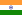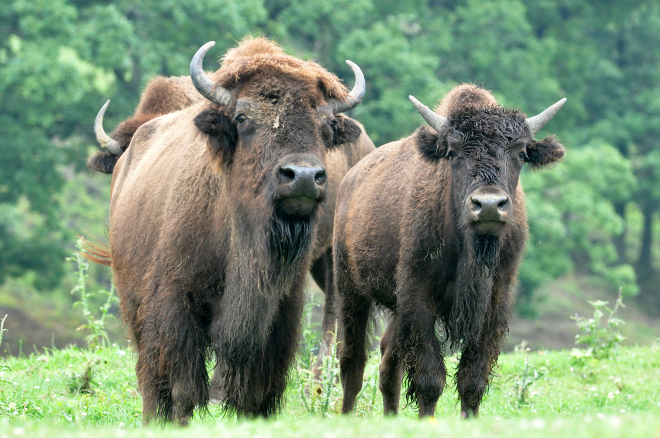# Buffallo Milk Production and Distribution With Good Returns

Opportunity Snapshot

• Country:India
• Industry: Agriculture
• Stage: Start-up
• Investment size: \$30,000 / min. \$10,000
• Type of investment: Debt, EquityThis is a project about Buffallo milk production and distribution starting with 100 buffallows which may produce at least 800 Lts. of milk per day. Image courtesy of Nedra, 2009, Flickr CC.

## Investment Opportunity

This is a project about Buffallo milk production and distribution starting with 100 buffallows which may produce at least 800 Lts. of milk per day.

The return of return ratio would be 50% of the net invested money.

No. of units (BUFALLOWS): 450
SPACE CONCERNING PER 10 UNIT: 20 Meter X 10 Meter = 200 Sq. Meter.
Total Unit Ground Sq. Required: 200 Sq. Meter x 450 = 90000 Sq. Meter.
Land Required in Acre: 25 Acres.
Cost of Land (Apprx.): 2 Crore.
Cost of units (Apprx.: 1.2 Crore. (Apprx. Minimum).
Total Investment: 3.5 Crore.

Total Expenditure per day/ unit :
1. Dry Grass: 20 Kg./ unit, 20 Kg. x 350 = Rs. 7000
2. Green Grass: 20 Kg./ unit, 20 Kg. x 350 = Rs. 5000
3. Cotton Brid (Khod): 05 Kg./ unit, 05 Kg. x 350 = 35 x 600 = Rs. 21000
4. Nutrition Powder : 02 Kg./ unit, 02 Kg. x 350 = 07 x 800 = Rs. 5600
5. Medicines: As required.
6. Workers : 60, 60 x 150 = Rs. 9000
Average Total Expense = Rs. 47600
Total Income per day/ unit:
Average capacity of milk by one unit = 6 Kg./ 12 Hrs. = 12 Kg./ day.
Average fat of milk per kg. = 6 to 7.5
Average price of fat = 3.5 to 3.75/fat
Average price of mild per kg. = Rs. 26.75
Average Total milk production/day = 12 x 350 = 4200 kg.
Average gross income/ day = 4200 kg. x 26.75 = Rs. 112350
Net Income per day = Total Income – Total Expenditure = Rs. 112350 - Rs. 47600 = Rs. 64750
1. Total Average Gross Income/ Month = Rs. 33, 70,500
2. Total Average Gross Expense/ Month = Rs. 14, 28,000
3. Total Average Extra Expense/ Month = Rs. 02, 00,000
(Medicines and other expenses)
Total Net Income/ Month = Rs. 17, 42,500
Total Net Income/ Year = Rs. 2, 09, 10000
Average Rate of Return/ Month = Total Income x 100
Total Investment = 1742500 x 100 = 35000000 = 4.978 %
Average Rate of Return/ Year = 4.978 x 12 = 59.76 % ∞ 60 %